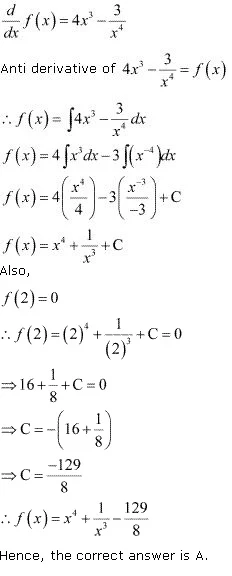# Class 12 Maths NCERT Solutions for Chapter 7 Integrals Exercise 7.1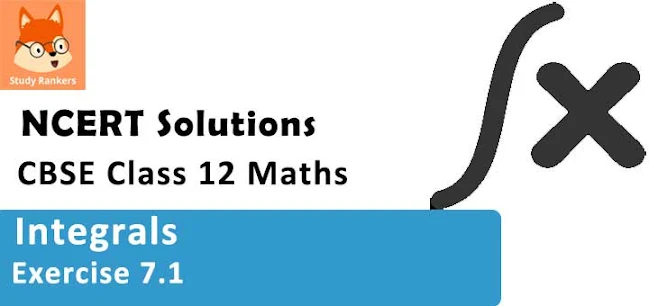### Integrals Exercise 7.1 Solutions

1. Find an anti derivative (or integral) of the following functions by the method of inspection.
sin 2x .

Solution

The anti derivative of sin 2x is a function of x whose derivative is sin 2x.
It is known that,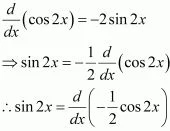Therefore, the anti derivative of sin2x is -1/2 cos 2x

2. Find an anti derivative (or integral) of the following functions by the method of inspection.
cos 3x .

Solution

The anti derivative of cos 3x is a function of x whose derivative is cos 3x.
It is known that,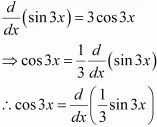Therefore, the anti derivative of cos3x is (1/3) sin 3x.

3. Find an anti derivative (or integral) of the following functions by the method of inspection.
e2x.

Solution

The anti derivative of e2is the function of x whose derivative is e2x.
It is known that,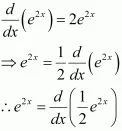Therefore, the anti derivative of e2x  is (1/2) e2x.

4. Find an anti derivative (or integral) of the following functions by the method of inspection.
(ax + b)2

Solution

The anti derivative of (ax + b)2 is the function of x whose derivative is (ax + b)2 .
It is known that,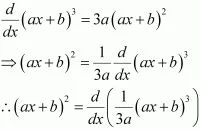Therefore, the anti derivative of (ax + b)2 is (1/3a)(ax + b)3.

5. Find an anti derivative (or integral) of the following functions by the method of inspection
sin 2x – 4 e3x

Solution

The anti derivative of (sin2x - 4e3x) is the function of x whose derivative is (sin 2x - 4e3x).
It is known that,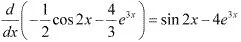Therefore, the anti derivative of (sin2x - 4e3x) is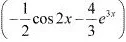6. Find the following integrals ∫(4 e3x + 1) dx

Solution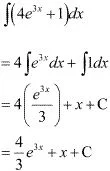7. Find the following integrals ∫[x2 (1 - 1/x2 ) dx
Solution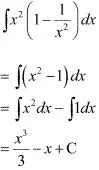8. Find the following integrals ∫(ax + bx + c) dx .
Solution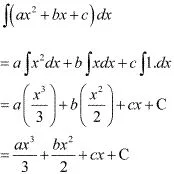9. Find the following integrals ∫(2x2 + ex ) dx .
Solution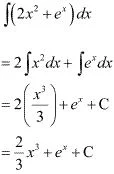10. Find the following integrals ∫ (√x - 1/√x)2 dx
Solution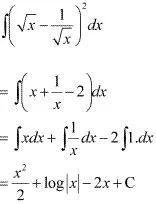11. Find the following integrals ∫[(x3 + 5x2 - 4)/x2 ] dx
Solution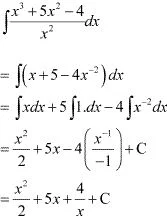12. Find the following integrals ∫[(x3 + 3x + 4)/√x] dx
Solution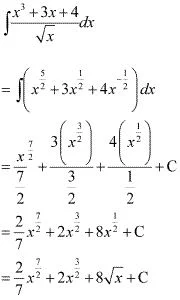13. Find the following integrals ∫[(x3 - x2 + x - 1)/(x - 1)] dx
Solution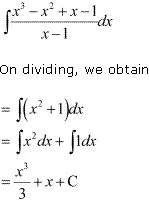14. Find the following integrals ∫(1 – x) √x dx
Solution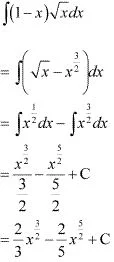15. Find the following integrals ∫ x( 3x2 + 2x + 3) dx
Solution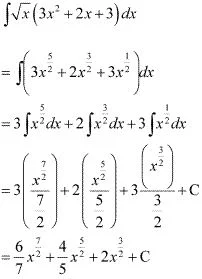16. Find the following integrals ∫(2x - 3 cos x + ex )dx
Solution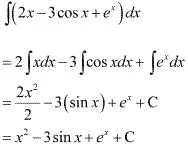17. Find the following integrals ∫(2x2 - 3 sin x + 5√x)dx
Solution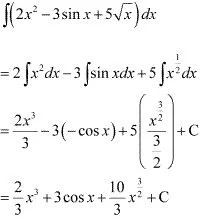18. Find the following integrals ∫sec x (sec x + tan x) dx
Solution
∫sec x(sec x + tan x)dx
= ∫(sec2 x + sec x tan x)dx
= ∫ sec2 x dx + ∫ sec x tan x dx
= tan x + sec x + c

19. Find the following integrals ∫ (sec2 x/cosec2 x) dx
Solution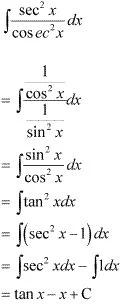20. Find the following integrals ∫ (2 - 3 sinx)/cos2 x  dx
Solution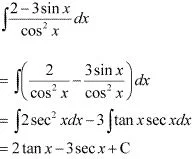21. The anti derivative of (√x + 1/x)equals
(A) (1/3) x1/3 + 2x1/2 + C
(B) (2/3)x2/3 + (1/2)x2 + C
(C) (2/3)x3/2 + 2x1/2 + C
(D) (3/2)x3/2 + (1/2)x1/2 + C
Solution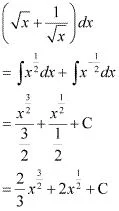Hence, the correct answer is C.

22.  If d/dx f(x) = 4x3 - 3/x4 such that f(2) = 0 then f(x) is
(A) x4 + 1/x3 - 129
(B) x3 + 1/x4 + 129/8
(C) x4 + 1/x3 + 129/8
(D) x3 + 1/x4 - 129/8
Solution
It is given that,